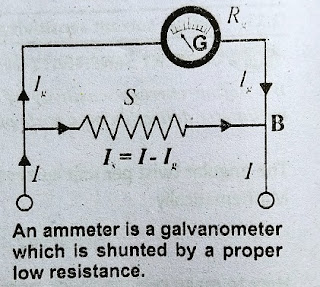Ammeter

Ammeter is an electrical device which is used to measure the current. It is the modified form of galvanometer. It is a low resistance galvanometer. It is always connected in series with circuit to measure current. An ideal ammeter has zero resistance.

Meter movement

The section of galvanometer whose motion give rise to the needle of the device to move across the scale is usually known as meter-movement.

Note

Mostly, the galvanometer gives full scale deflection even when a few milliampere current passes through it. So for large current measurement, it should be modified.

CONVERSION OF GALVANOMETER INTO AMMETER

shunt (bypass) resistor

A galvanometer can be converted into ammeter by connecting a low resistance Rs called shunt (bypass) resistor in parallel with the meter- movement (coil) of galvanometer.

Range of Ammeter

Let, I be the range of ammeter

Calculation of Shunt Resistance

Shunt resistance provides another path for flow of current. The value of Rs is so adjusted that the current which gives full scale deflection passes through galvanometer and ramaining current passes through Rs.

Let,

Resistance of galvanometer = Rg

Full scale deflection current = Ig

The current passes through Rs = I - Ig

Now potential difference across the Rg is,

Vg = Ig Rg 𑁋(1)

Similarly potential difference across the Rs is,

Vs = Is Rs

OR Vs (I - Ig) Rs 𑁋(2). [Since Is = I - Ig]

Since the galvanometer resistance Rg and the shunt resistance Rs are connected in parallel, so the potential difference across them must be same i.e.,

Vs = Vg

Putting values, we get

(I - Ig) Rs = Ig Rg

Or Rs = Ig Rg / I - Ig  𑁋(3)

Note

The value of shunt Rs is very small. We can use a copper wire for this purpose which should be connected parallel across the galvanometer. In order to increase the range of ammeter Rs should be decreased.